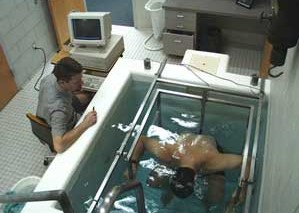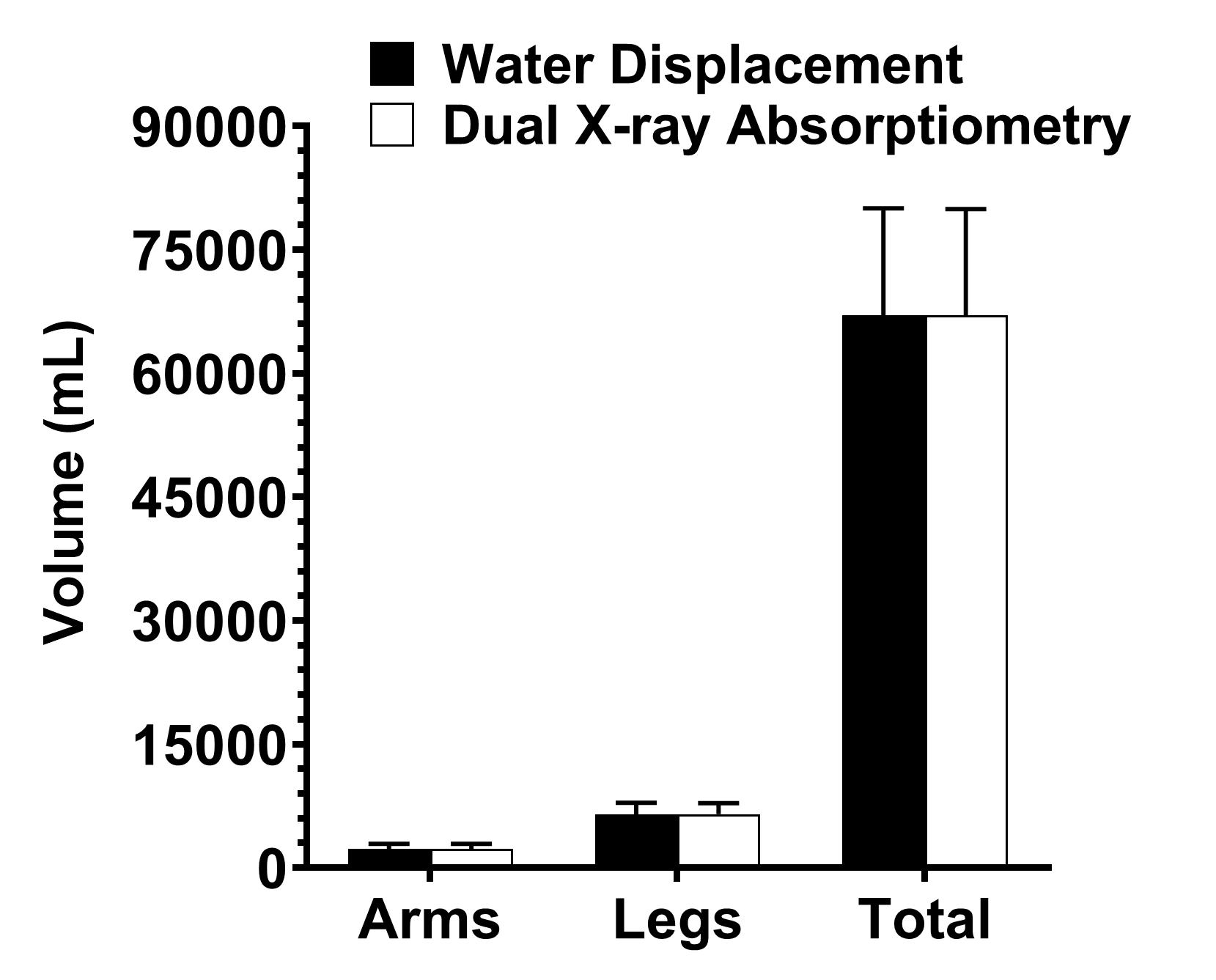We recently published an article in Clinical Nutrition ESPEN (Czeck et al., 2022) on a research study that we conducted to evaluate the accuracy of using dual X-ray absorptiometry (DXA) to determine total as well as regional body volumes. You may ask, “What would be the value of the DXA being able to determine volume(s)?” Well, one reason would be that determining volume by DXA would allow one to use DXA, in conjunction with a device such as bioelectrical impedance (BIA) or bioelectrical spectroscopy (BIS), to determine body composition using a four-compartment model. Using body water from BIA or BIS, with the three compartments determined by DXA (i.e., lean mass, bone mass, fat mass) and DXA-derived volume, you would be able to develop a convenient four-compartment model of body composition.  Since DXA can make regional as well as total measures, you would be able to make regional determination of body composition using a four-compartment model. This would increase the accuracy of your total as well as regional measure of body composition.  This accurate measure of regional body composition could be useful in evaluating disease, intervention, performance, injury risk, and rehabilitation.

Before we can use DXA in a four-compartment model of body composition, we first need to know if it can accurately determine volume.  For total body volume, this is relatively simple.  If you have an underwater weighing system (Figure 1) you can easily determine the volume of the total body by water displacement.  However, for an individual limb (i.e., leg, arm) the same underwater weighing tank that is used to measure total body volume is too large for regional measures.  So, to make accurate measurements of limb volume we used specially constructed tanks (Figure 2). We then compared these regional measures of arm and leg volume as well as total body volume to DXA-derived region of interest boxes for arm and leg volumes as well as total body volume. What we found was that DXA-derived measures of total body volume were not significantly different from total body volume measured via total body water displacement (Figure 3). In addition, both arm and leg regional DXA-derived volumes were not significantly different than regional volumes measured by regional water displacement (Figure 3).(Figure 1)(Figure 2)(Figure 3)

Using this data we were able to construct a formula to estimate total body volume (milliliters) from DXA (see below):

Total body volume (mL) = 1.12 x fat mass (kg) + 0.94 x lean mass (kg) – 1.17 x bone mineral content (kg) - 783.7 (mL)

Again, using this data we were able to construct a formula to estimate arm volume (milliliters) from DXA (see below):

Arm volume (mL) = 0.66 x fat mass (kg) + 0.68 x lean mass (kg) + 1.40 x bone mineral content (kg) + 311.99 (mL)

Finally, using this data we were able to construct a formula to estimate leg volume (milliliters) from DXA (see below):

Leg volume (mL) = 1.13 x fat mass (kg) + 0.83 x lean mass (kg)  + 1.04 x bone mineral content (kg) – 24.96 (mL)

TAKE-HOME MESSAGE

You might be wondering, what does this research really mean? Well, for starters, there were no significant differences between total and regional DXA-derived volumes compared to a traditional method used for actually measuring volume. Suggesting that DXA can be used to estimate not only total body volume, but regional (arm, leg) volumes as well. The results of this study expand the DXA's capabilities and potentially allow for a convenient regional four-compartment model with DXA-derived regional volumes to be developed. No longer do you need specially designed water tanks to determine volume. Therefore, coupling DXA with BIS would allow one to develop a regional four-compartment model. This four-compartment model could be used to determine regional changes in body composition due to cancers, tumors, or trauma which result in the volumetric loss of muscle. It could also be used in athletes to more accurately determine total as well as regional body composition. Future studies are needed to explore the DXA-derived regional volume in a variety of populations and its utility in the development of regional four-compartment models of body composition.

REFERENCES

Czeck MA, Roelofs EJ, Juckett WJ, Dengel DR: Dual X-ray absorptiometry-derived total and regional body volume. Clinical Nutrition ESPEN 52:100-104, 2022.